# 如何將 TensorFlow 迴歸模型透過 TFLite 部署在 Android 裝置

2021/09/02

## 前言

TensorFlow Lite 使用更小的位元大小以及輕量運算量得到更好的效能，對行動裝置以及嵌入式裝置更加的輕量化。佈署 TensorFlow Lite 的時候，首先要把 Python 訓練好的模型透過 TensorFlow Lite Converter 進行轉換成 `.tflite`，就可以將轉換好的模型部署在行動裝置中。

• TFLite：基於 FlatBuffers 對模型進行優化，可以直接部署到 Android、iOS 等終端設備上。

## 本文中您將學到

• 使用 Python TensorFlow Kears API 訓練一個簡單的迴歸 NN 模型
• 模型的儲存
• 將儲存的模型打包成 `.tflite`
• 將 TFLite 模型放到 Android 手機上
• 使用 Interpreter 測試 TFLite 模型

## TensorFlow

TensorFlow 是由 Google 所開源的深度學習套件。它可以被執行在許多種平台上，例如網站或是行動裝置甚至是嵌入式IoT裝置。然而訓練好的 Tensorflow 模型並不能直接部署到這些設備上，必須透過轉換器將模型轉成 `tflite`## 建立資料集

``````import numpy as np

X = np.arange(1, 500, 1)
y = np.arange(2, 1000, 2)
``````

## 搭建神經網路模型

Keras 有三種模型搭建的方式，詳細內容可以參考這篇文章。在本範例中使用大家最常見的 Sequential 搭建一層神經網路，其中神經元僅有一個，另外沒有設定 `activation` 預設即為 `linear`。我們也能夠過 `model.summary()` 查看模型描述，可以發現模型有兩個權重分別為 w 與 bias。

``````from tensorflow import keras
from tensorflow.keras import Sequential
from tensorflow.keras.layers import Dense

model = Sequential([Dense(1, input_shape=)])
model.fit(X, y, epochs=100)
``````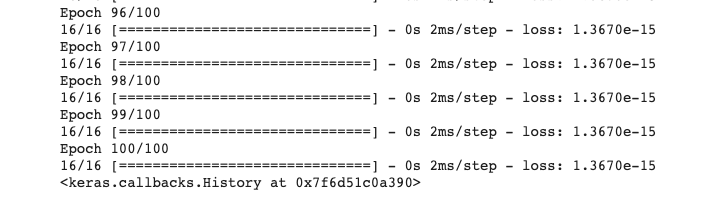## 測試模型

``````model.predict()
``````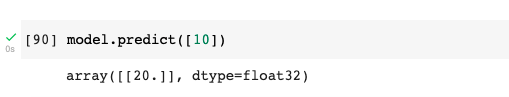## 儲存模型

``````model.save('regression.h5')
``````

``````import tensorflow as tf
converter =tf.lite.TFLiteConverter.from_keras_model(model)
converter.optimizations = [tf.lite.Optimize.DEFAULT]
tflite_model = converter.convert()
open("regression.tflite", "wb").write(tflite_model)
``````## TFLite Interpreter

``````import tensorflow as tf
import numpy as np

interpreter = tf.lite.Interpreter(model_path='regression.tflite')
interpreter.allocate_tensors()

input_index = interpreter.get_input_details()["index"]
output_index = interpreter.get_output_details()["index"]

input_data = np.array([], dtype=np.float32)
interpreter.set_tensor(input_index, input_data)
interpreter.invoke()

print(interpreter.get_tensor(output_index))
``````

## 將 TFlite 模型部署到 Android 手機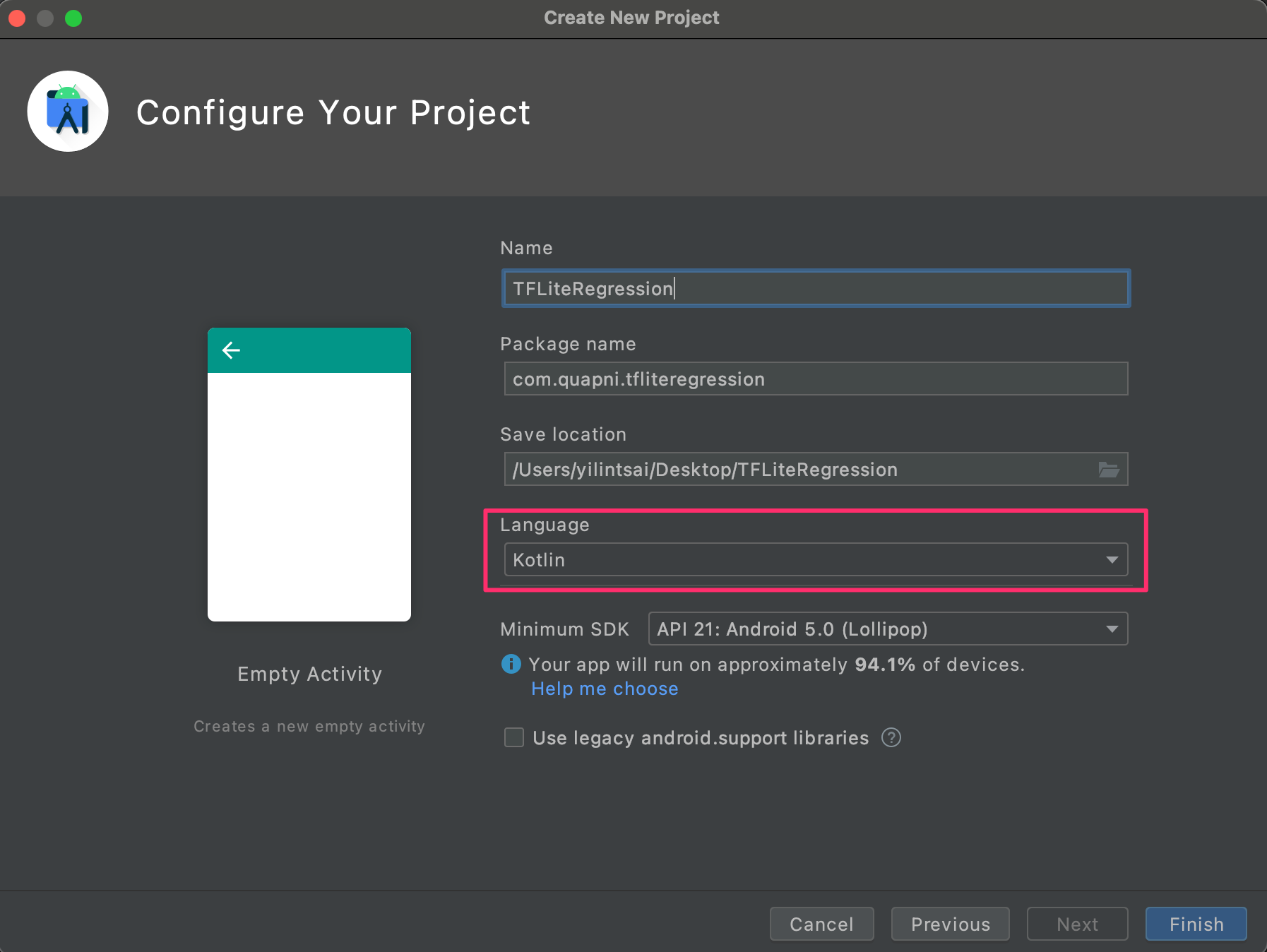``````implementation "org.tensorflow:tensorflow-lite:+"
``````

``````aaptOptions {
noCompress "tflite"
noCompress "lite"
}
``````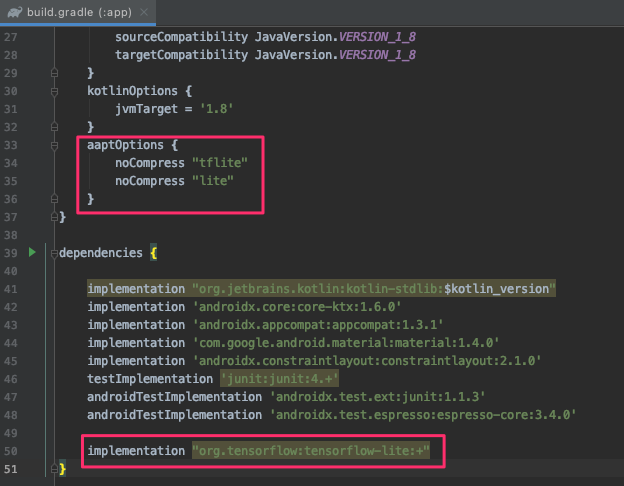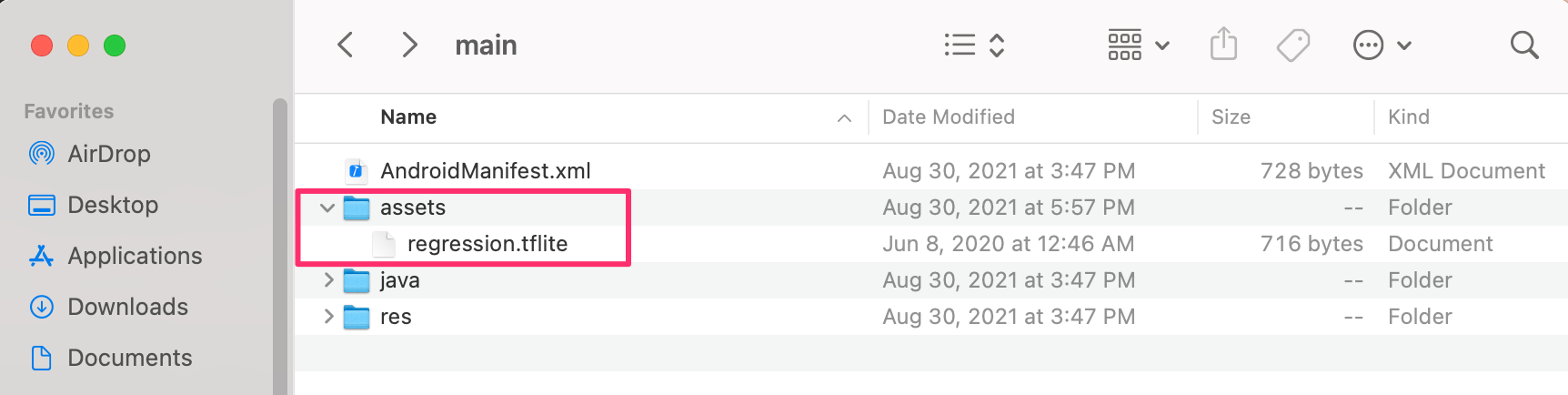``````<?xml version="1.0" encoding="utf-8"?>
<androidx.constraintlayout.widget.ConstraintLayout xmlns:android="http://schemas.android.com/apk/res/android"
xmlns:app="http://schemas.android.com/apk/res-auto"
xmlns:tools="http://schemas.android.com/tools"
android:layout_width="match_parent"
android:layout_height="match_parent"
tools:context=".MainActivity">

<EditText
android:id="@+id/numberField"
android:layout_width="wrap_content"
android:layout_height="wrap_content"
android:ems="10"
android:inputType="number"
app:layout_constraintBottom_toTopOf="@+id/guideline"
app:layout_constraintEnd_toEndOf="parent"
app:layout_constraintStart_toStartOf="parent" />

<androidx.constraintlayout.widget.Guideline
android:id="@+id/guideline"
android:layout_width="wrap_content"
android:layout_height="wrap_content"
android:orientation="horizontal"
app:layout_constraintGuide_percent="0.5" />

<Button
android:id="@+id/btnPredict"
android:layout_width="wrap_content"
android:layout_height="wrap_content"
android:layout_marginTop="16dp"
android:text="Predict"
app:layout_constraintEnd_toEndOf="@+id/numberField"
app:layout_constraintStart_toStartOf="@+id/numberField"
app:layout_constraintTop_toBottomOf="@+id/numberField" />

<TextView
android:id="@+id/txtResult"
android:layout_width="wrap_content"
android:layout_height="wrap_content"
android:layout_marginTop="32dp"
android:fontFamily="sans-serif-black"
android:textColor="@android:color/black"
android:textSize="18sp"
app:layout_constraintEnd_toEndOf="@+id/btnPredict"
app:layout_constraintStart_toStartOf="@+id/btnPredict"
app:layout_constraintTop_toBottomOf="@+id/btnPredict" />
</androidx.constraintlayout.widget.ConstraintLayout>
``````

``````class MainActivity : AppCompatActivity() {

private lateinit var interpreter: Interpreter
private val mModelPath = "regression.tflite"

private lateinit var resultText : TextView
private lateinit var editText : EditText
private lateinit var checkButton : Button
override fun onCreate(savedInstanceState: Bundle?) {
super.onCreate(savedInstanceState)
setContentView(R.layout.activity_main)

resultText = findViewById(R.id.txtResult)
editText = findViewById(R.id.numberField)
checkButton = findViewById(R.id.btnPredict)

checkButton.setOnClickListener {
var result = doInference(editText.text.toString())
resultText.text = result.toString()
}
}

initInterpreter()
}

private fun initInterpreter(){
val options = Interpreter.Options()
options.setUseNNAPI(true)
}
private fun doInference(inputString: String): Float {
val inputVal = FloatArray(1)
inputVal = inputString.toFloat()
val output = Array(1) { FloatArray(1) }
interpreter.run(inputVal, output)
return output
}

private fun loadModelFile(assetManager: AssetManager, modelPath: String): MappedByteBuffer {
val fileDescriptor = assetManager.openFd(modelPath)
val inputStream = FileInputStream(fileDescriptor.fileDescriptor)
val fileChannel = inputStream.channel
val startOffset = fileDescriptor.startOffset
val declaredLength = fileDescriptor.declaredLength
}
}
``````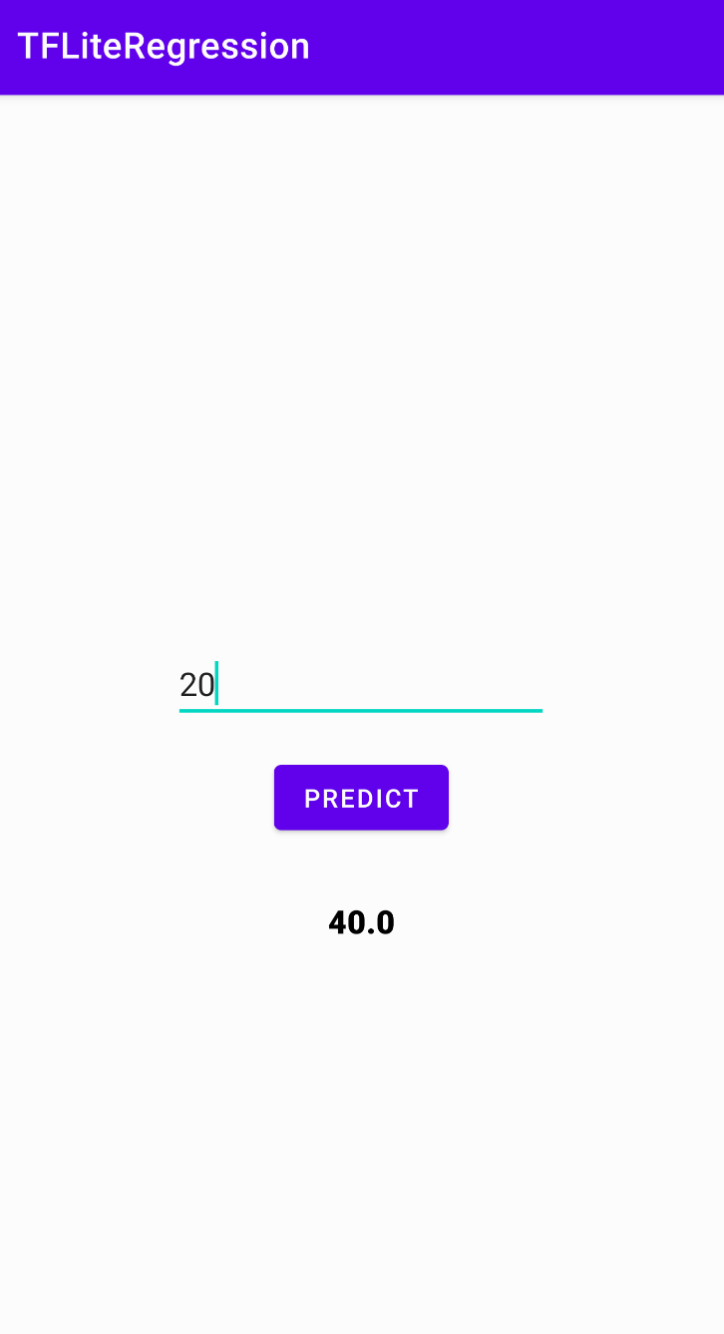## Referencehttps://www.youtube.com/channel/UCSNPCGvMYEV-yIXAVt3FA5A